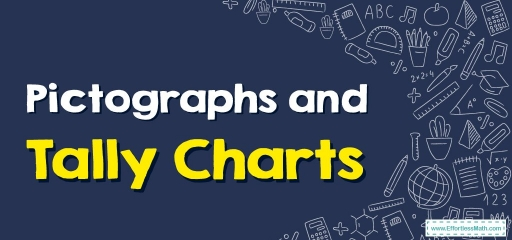# Pictographs and Tally Charts## What’s a Tally Chart?

Tally charts are tables utilized to count and compare the amounts of several classes on a data set.

These tally charts are utilized to gather information swiftly and effectively since putting marks on a chart is much faster than putting down words. This format similarly gives itself well to accumulating information in sub-groups and that could be quite valuable.

The preliminary data gets recorded via the use of tally marks or ‘hashes,’ arranged into groups of $$5$$. That makes it much simpler to keep track of the full total because you merely count groups of these tally marks by $$5$$.

## Related Topics

Tally charts are merely one way to collect info via tally marks. The information then can be utilized to generate graphs with more details as well as visual graphs and charts used to do a comparison of the info you gathered.

Normally, whenever we’ve completed tally charts, we will go on to denote their information via a pictogram.

Pictograms are charts utilizing pictures for representing the information. Pictograms get set out exactly the precise way bar charts are, although as an alternative to bars they utilize columns of images to denote the involved numbers.

## Key to a Pictograph

You utilize a key to signify a symbol’s value. In the example above, the key was one icon of the pizza signifying four kids.

• All the used symbols/icons have to be exactly the precise equal size.
• A small percentage of an icon can likewise be utilized to denote the data.

Therefore, after seeing the previous pictograph example, you get the basic formula used to get the data’s value for the categories shown on the table/chart.

Value for a category $$=$$ Product of the number of times the symbol gets utilized as well as the value of each of the symbols. If you show the number of times by ‘$$N$$’, as well as the value of each of the symbols by ‘$$S$$’, you end up with:

Category’s value $$= N × S$$

## Way to Create a Pictograph

The steps below can be used to correspond to the information provided in a table by using the format of a pictograph.

Gather Data: First, you must gather the info for several various categories you wish to show, then create a list or a table.

Go through this info, then choose your symbol: Go over the gathered info, and based on this choose a picture or a symbol that portrays this info accurately.

Designate a Key: Dependent on the rate of the information, determine the rate for a symbol. That is possible via designating a mathematical value to which a symbol will correspond. That key or mathematical value has to be written alongside your pictograph.

Draw a pictograph: Ultimately, draw your pictograph via primarily drawing $$2$$ columns representing your category as well as your info. Afterward, draw the real symbols that represent the rates. You can draw the symbols as fractions too if the rate isn’t a whole number or built on your key.

Review the Pictograph: Review your labels as well as the pictograph and then ensure it signifies the info correctly.

### Pictographs and Tally Charts – Example:

Using the key, draw the pictograph to show the information.

Solution:

$$2$$ apples = $$1$$ star. We have $$12$$ lines in this chart, so $$12$$ divided by $$2$$: $$\frac{12}{2}=6$$ We have to draw $$6$$ stars.

## Exercises for Pictographs and Tally Charts

Using the key, draw the pictograph to show the information.

1)

2)

1)

2)

### What people say about "Pictographs and Tally Charts - Effortless Math: We Help Students Learn to LOVE Mathematics"?

No one replied yet.

X
30% OFF

Limited time only!

Save Over 30%

SAVE $5 It was$16.99 now it is \$11.99